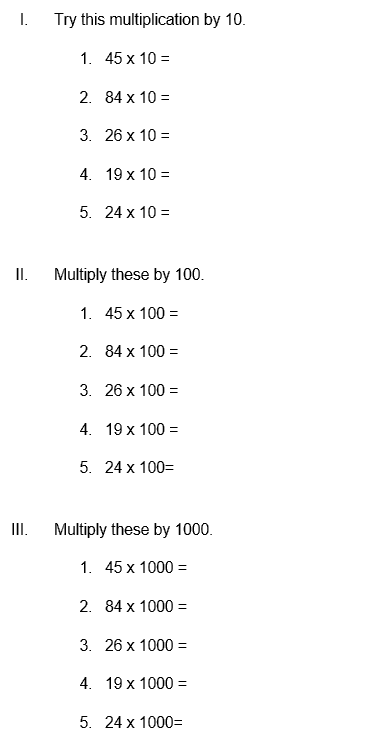# Multiply by 10, 100, and 1000 Worksheet (with answer key + PDF)

If a whole number is multiplied by 10, a 0 is added at the end. If a whole number is multiplied by 100, two zeros are added at the end. If a whole number is multiplied by 1000, three zeros are added at the end.

## What is the “Multiply by 10, 100, and 1000 Worksheet”

This worksheet will explore multiplication primarily by 10, 100, and 1000.

## What is Multiply by 10, 100, and 1000?

When multiplying a number by 10, 100, or 1000, we must count the zeroes in the multiplier and add the same number to the multiplicand’s right.

How will the “Multiply by 10, 100, and 1000 Worksheet” help you?

This worksheet will help the kids to solve multiplication. Also, this worksheet will discuss how to solve multiplication problems.

## Instructions on how to use the “Multiply by 10, 100, and 1000 Worksheet.”

Study the concept and examples given and try to solve the given exercises below.

## Conclusion

All the digits are shifted to the left when multiplying by multiples of 10, 100, and 1000. Ten is the only number left, followed by one hundred, two hundred, and three thousand. In contrast, we shift all the digits to the right when dividing by 10, 100, and 1000.

If you have any inquiries or feedback, please let us know.

## Multiply by 10, 100, and 1000 Worksheet (with answer key + PDF)

• If we multiply a whole number by a 10, then we write one zero at the end of the multiplicand.

For example:

1275 × 10 = 12750

• If we multiply a whole number by 100, then we write two zeros at the end of the multiplicand.

For example:

1275 × 100 = 127500

• If we multiply a whole number by 1000, then we write three zeros at the end of the multiplicand.

For example:

1275 × 1000 = 1275000

• To multiply a number by a multiplier having zero and non-zero parts, we put as many zeroes in the product as in the multiplier and then multiply the number by the non-zero part.

For example:

1275 × 20 = 25500

1275 × 300 = 382500

1275 × 5000 = 6375000

## Worksheet